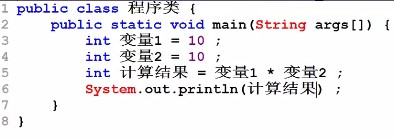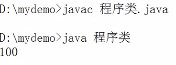Java零基础课程笔记（三）——Java数据类型划分

1. 数据类型划分

1.1 java数据类型划分（牢记）

• byte：-128~127
• int：-2147483648~2147483647

• 在程序开发中，整数选择int，描述小数使用double
• long一般描述日期时间、内存或文件大小（字节）
• 若需要进行编码转换或进行二进制传输，使用byte
• char一般在描述中文的时候使用
• boolean在描述程序逻辑时使用

1.2 整型

  在Java程序中任何一个整数的常量类型都是整型。


public class Test{
public static void main(String args[]){
//10是一个整数（不会改变的数据是常量）
//程序之中任何一个整数对应的类型都是int
int num= 10;//声明一个int型变量
//num是in图形变量*2是int型常量，int*int还是int
System.out.println(num*2);
}
} 

public class Test{
public static void main(String args[]){
int maxValue =  Integer.MAX_VALUE;//取得最大值
int minValue = Integer.MIN_VALUE;//取得最小值
System.out.println(maxValue);//2147483647
System.out.println(minValue);//-2147483648
System.out.println(maxValue+1);//-2147483648数据溢出为最小值
System.out.println(minValue -1);//2147483647数据溢出为最大值
}
} 
• int 和 Integer区别简述：为了编程的方便引入了基本数据类型，但是为了能够将这些基本数据类型当成对象操作，Java为每 一个基本数据类型都引入了对应的包装类型（wrapper class），int的包装类就是Integer。int 是基本类型，直接存数值，进行初始化时int类的变量初始为0。Integer是对象，用一个引用指向这个对象，Integer的变量则初始化为null。

public class Test{
public static void main(String args[]){
int maxValue =  Integer.MAX_VALUE;//取得最大值
int minValue = Integer.MIN_VALUE;//取得最小值
System.out.println(maxValue);//2147483647
System.out.println(minValue);//-2147483648
long result = maxValue + 1;
System.out.println(result);//-2147483647
}
} 

• 直接将int变量的内容复制给long变量

      long result = maxValue;//将int的数据变量赋值给了long
//result为long，+1为int，long+int=long
System.out.println(result+1);//2147483648
• 直接将某一个int型的变量变为long型

public class Test{
public static void main(String args[]){
int maxValue =  Integer.MAX_VALUE;//取得最大值
int minValue = Integer.MIN_VALUE;//取得最小值
long result = (long)maxValue + 1;
System.out.println(result);//2147483648
}
} 

public class Test{
public static void main(String args[]){
// long num = 2147483648;
// 任何整数其默认类型都是int，但该数据已经超过了今天的范围
// 那么现在需要将这个数据明确的表示是一个long型的常量，在尾部加上L或l。
long num = 2147483648L;
// 在强制的时候设置其目标类型，这种操作有可能会丢失精度。
int result = (int) num;//大类型变小类型，必须强制
System.out.println(result);//-2147483647
}
} 

+ 范围小的数据类型可以自动变换为范围大的数据类型
+ 范围大的数据类型变为范围小的数据类型需要进行强制转换

说明：关于数据类型默认值问题

public class Test{
public static void main(String args[]){
int num;//定义一个num变量
System.out.println(num);//编译出错
}
} 

• 在使用前进行赋值（在老版本JDK会出错）
代码
public class Test{
public static void main(String args[]){
int num;//定义一个num变量
num = 10;在num输出之前为其赋值
System.out.println(num);//输出10
}
} 
• 在定义变量时进行赋值
代码
public class Test{
public static void main(String args[]){
int num = 10;//定义一个num变量，同时初始化
System.out.println(num);//输出10
}
} 
byte数据类型（-128~127）： 范例：声明byte

public class Test{
public static void main(String args[]){
byte data  = 10;
//10是int，int的范围大于byte，理论上需要强制类型转换，但这里没有转换，因为其数据量符合byte要求
System.out.println(data);//输出10
}
} 

public class Test{
public static void main(String args[]){
/*   byte data  = 128;
//大于byte
System.out.println(data);//错误：不兼容数据类型
*/
byte data  = (byte) 128;
//大于byte,强转
System.out.println(data);//-128
}
} 
注意如果要将int常量直接赋给byte变量，那么只能是int常量，不能是int变量。

public class Test{
public static void main(String args[]){
int num = 10;
byte data  = (byte) num;
System.out.println(data);//10
}
} 

1.3 浮点类型

public class Test{
public static void main(String args[]){
double num = 10.2;// 这是一个小数，默认类型为double
//num是double型 * 2 int型 = double型
// num * 2.0(由nt变为的double型)
System.out.println(num * 2);//-128
}
} 
double可以保存的数字式最大的，也就是说double可以保存全宇宙中最大的有意义的数，除了double外也可用float描述小数，数据范围double>float，若直接声明float变量，要求在定义时进行强制转换：需要给数字后加上F或f，或在数字前加(float)，如float f = 2.23f; float f = (float) 2.23;。

public class Test{
public static void main(String args[]){
float f1 = 1.1f;
// float型*int常量 = float型
System.out.println(f1 * 2);// 2.2
System.out.println(1.1 * 1.1);//1.21000000000000002，传统bug
}
} 

public class Test{
public static void main(String args[]){
int a = 10;
int b = 4;
// int / int = int
System.out.println(a/b); // 2,若要进行准确运算，则必须保证有一个double型或float型
System.out.println(a/(double)b); // 2.5
}
} 

1.4 字符型

public class Test{
public static void main(String args[]){
char c = 'A';// 定义字符
System.out.println(c); // A
}
} 

public class Test{
public static void main(String args[]){
char c = 'A';// 定义字符
int num = c;// 将字符变为int型
System.out.println(num); // 65
}
} 

• 大写字母（A-Z）：65(A)~90(Z)
• 小写字母（a-z）：97(a)~122(z)
• 字符数字(‘0’-‘9’)：48(‘0’)~57(‘9’)

public class Test{
public static void main(String args[]){
char c = 'A';// 定义字符
// char + int = int
int num = c + 32;// 将字符变为int型
System.out.println(c); // A
System.out.println(num); // 97
// int转char需要强制类型转换
char x = (char) num;
System.out.println(x); // a
}
} 

public class Test{
public static void main(String args[]){
char c = '周';
int num = c;
System.out.println(num); // 21608
int num2 = c + 32;
System.out.println((char) num2); //咈
}
}这样就打破了命名标识符，但是不要用。

1.5 布尔型

public class Test{
public static void main(String args[]){
boolean flag = 10 > 2;// true
if (flag) {// if语句需要使用布尔条件
System.out.println("true！"); //true！
}
}
} 

1.6 初见字符串

char可以描述的只是单一的字符，但现在若想描述出多个字符，就必须利用字符串这一概念解决。例如定义表示人姓名的变量，很明显是多个字符，就必须使用String来描述。但是需要说明的是，String并不是基本数据类型，他是一个引用数据类型，但它的特殊在于可以使用基本数据类型的使用模式来进行套用。

• String变量上也可以使用‘+’，一旦使用了‘+’则描述的是两个个字符串的连接

public class Test{
public static void main(String args[]){
String name = "lala";//定义了一个String
String note = "qlql";//定义了一个String
System.out.println(name + note); // lalaqlql
}
} 

加号一般表示数学计算，但在String中表示字符串的连接，如果两类情况同时出现呢？

public class Test{
public static void main(String args[]){
int numA = 10;
double numB = 20.1;
//此时不是数学计算，而是连接
String result = "数学计算：" + numA + numB ;
System.out.println(result); // 数学计算：1020.1
/*
String result = "数学计算：" + numA - numB ;
System.out.println(result); // 编译出错
*/
}
} 

public class Test{
public static void main(String args[]){
int numA = 10;
double numB = 20.1;
//此时不是数学计算，而是连接
String result = "数学计算：" +( numA + numB );
System.out.println(result); // 数学计算：30.1
}
} 

2 总结

1.现阶段所考虑的数据类型为：int ，double，boolean；
2.操作的数据请保证有意义，就刻意回避数据范围的问题；
3.使用"声明的为字符串，字符串使用String来定义类型。